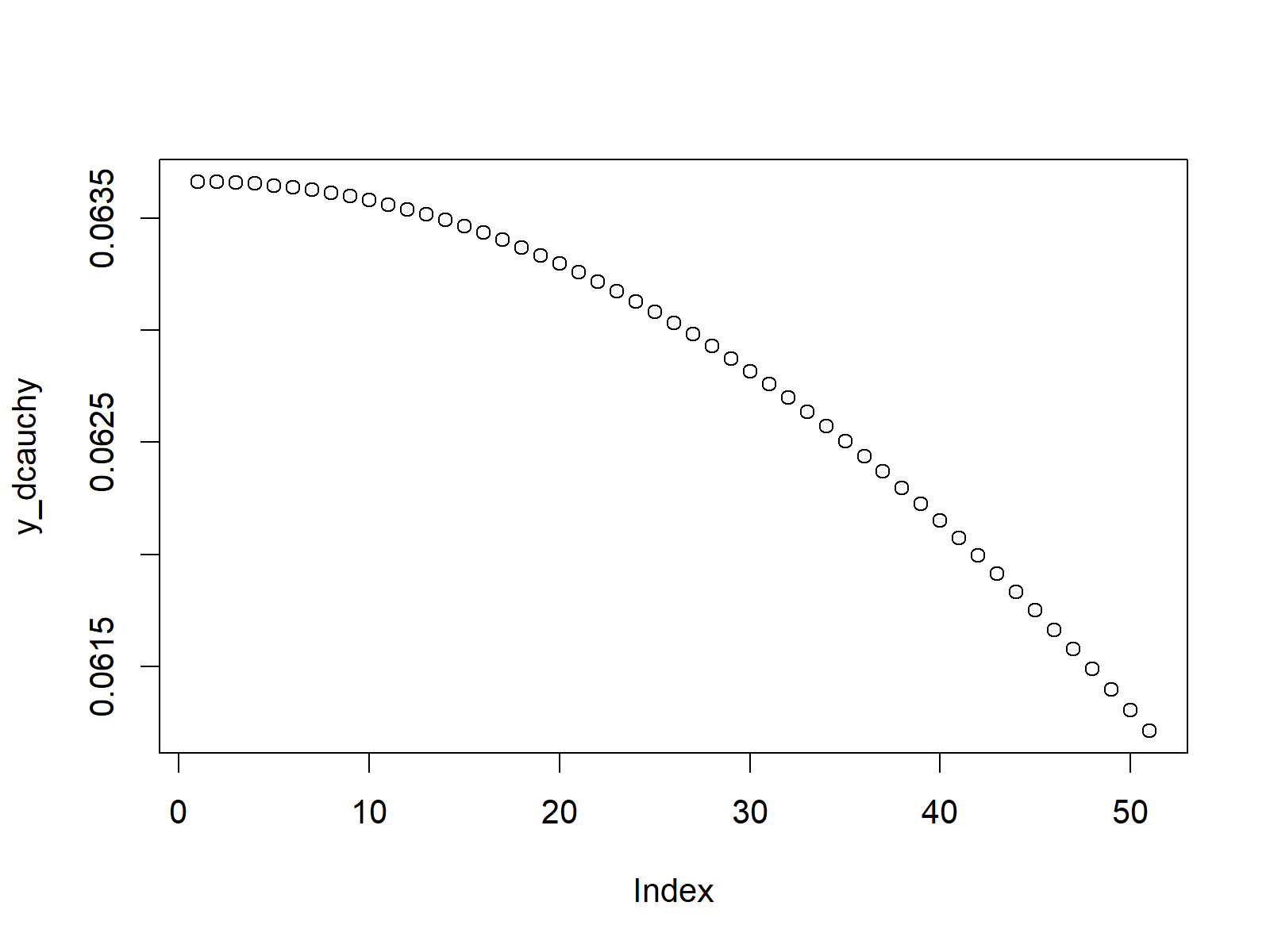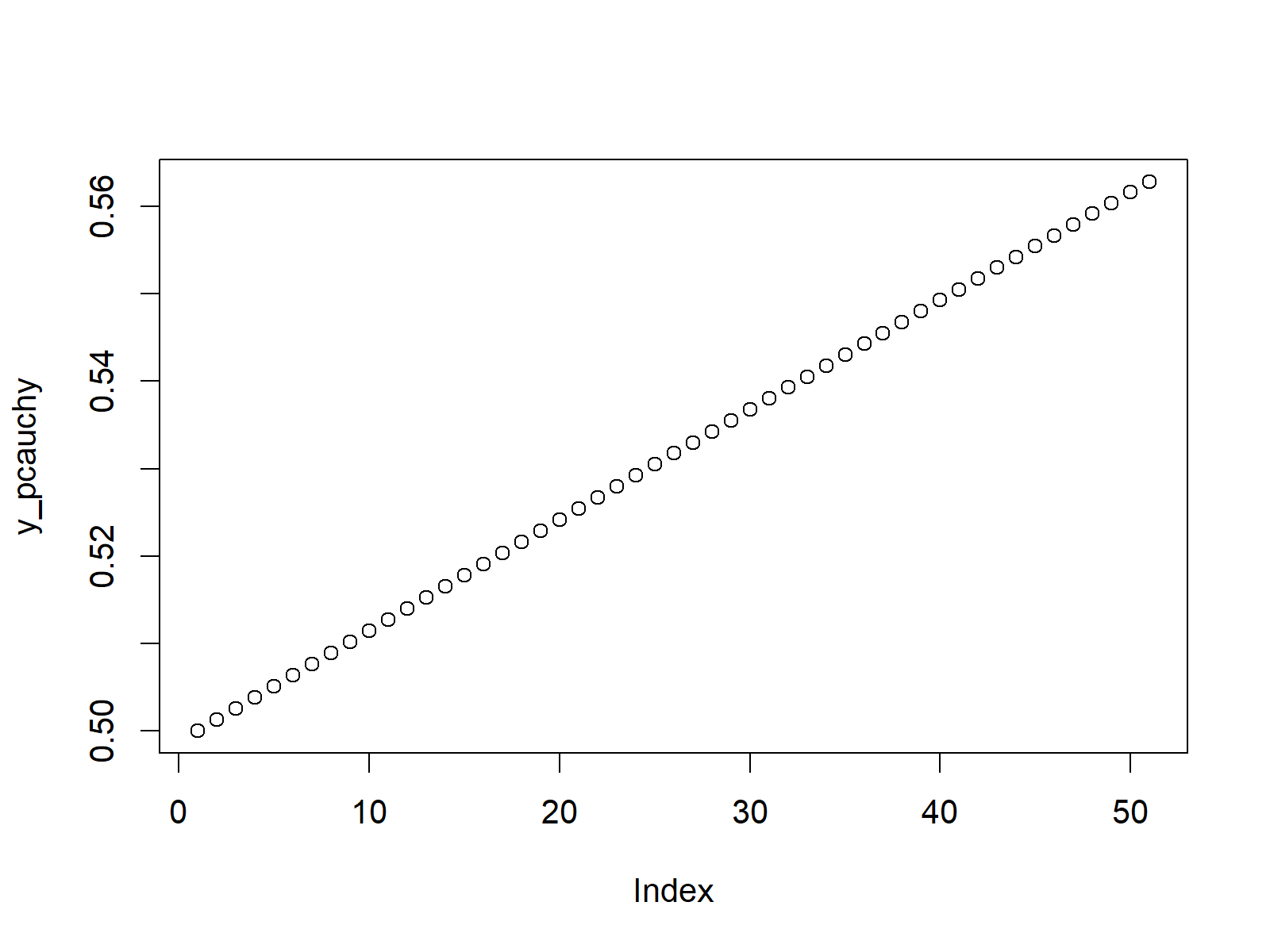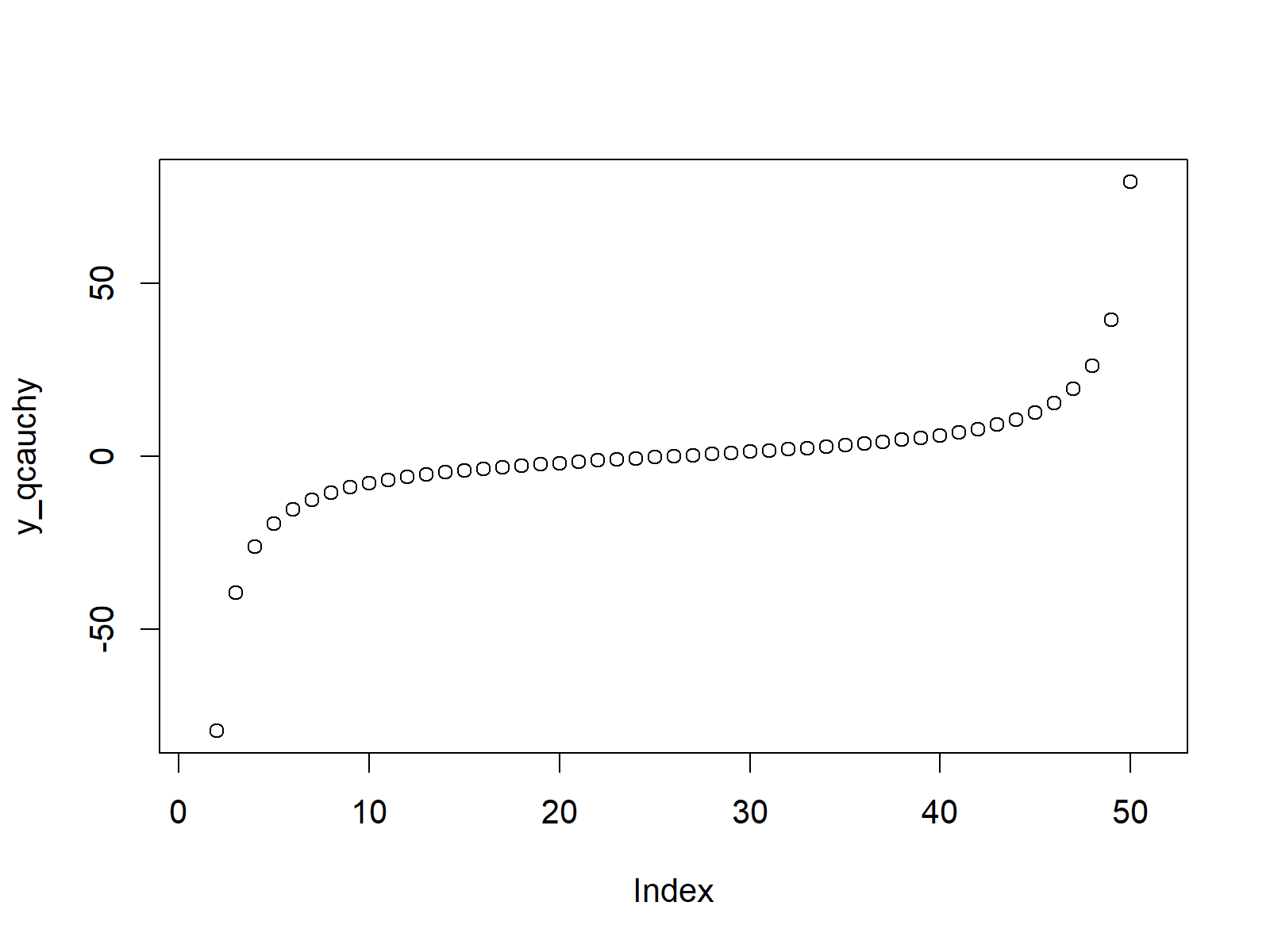# Cauchy Density in R (4 Examples) | dcauchy, pcauchy, qcauchy & rcauchy Functions

In this R tutorial you’ll learn how to apply the cauchy functions.

The page contains four examples for the application of dcauchy, pcauchy, qcauchy, and rcauchy. More precisely, the article will consist of this content:

Let’s get started:

## Example 1: Cauchy Density in R (dcauchy Function)

In Example 1, I’ll show you how to create a density plot of the cauchy distribution in R. First, we need to create an input vector containing quantiles:

`x_dcauchy <- seq(0, 1, by = 0.02)                     # Specify x-values for cauchy function`

Now, we can apply the dcauchy R function to return the values of a cauchy density. In the examples of this tutorial, we use a scale of 5. However, you could modify the R syntax according to your specific preferences.

`y_dcauchy <- dcauchy(x_dcauchy, scale = 5)            # Apply dcauchy function`

Our cauchy density values are now stored in the data object y_dcauchy. If we want to draw a density plot based on these values, we can use the plot function as shown below:

`plot(y_dcauchy)                                       # Plot dcauchy values`Figure 1: Cauchy Density in R.

## Example 2: Cauchy Cumulative Distribution Function (pcauchy Function)

Example 2 shows how to draw a plot of the cumulative distribution function (CDF) of the cauchy distribution. As a first step, we need to create a vector of quantiles:

`x_pcauchy <- seq(0, 1, by = 0.02)                     # Specify x-values for pcauchy function`

We can now apply the pcauchy R function to get the cauchy CDF values of our input vector:

`y_pcauchy <- pcauchy(x_pcauchy, scale = 5)            # Apply pcauchy function`

The final graphic can be created as follows:

`plot(y_pcauchy)                                       # Plot pcauchy values`Figure 2: Cumulative Distribution Function of Cauchy Distribution.

## Example 3: Cauchy Quantile Function (qcauchy Function)

The qcauchy command takes an input vector of probabilities and returns the corresponding values of the cauchy quantile function. Consider the following input vector:

`x_qcauchy <- seq(0, 1, by = 0.02)                     # Specify x-values for qcauchy function`

Now, the qcauchy function is applied as follows…

`y_qcauchy <- qcauchy(x_qcauchy, scale = 5)            # Apply qcauchy function`

…and the corresponding scatterplot is created as follows:

`plot(y_qcauchy)                                       # Plot qcauchy values`Figure 3: Quantile Function of Cauchy Distribution.

## Example 4: Random Number Generation (rcauchy Function)

We can also simulate random numbers that are distributed as the cauchy density. First, we need to specify a seed to ensure reproducibility and a sample size that we want to generate:

```set.seed(13579)                                       # Set seed for reproducibility
N <- 10000                                            # Specify sample size```

Now, we can apply the rcauchy function to create a set of random values as follows:

```y_rcauchy <- rcauchy(N, scale = 5)                    # Draw N cauchy distributed values
y_rcauchy                                             # Print values to RStudio console```

A histogram of our random data can be created as follows:

```hist(y_rcauchy,                                       # Plot of randomly drawn cauchy density
xlim = c(- 200, 200),
breaks = 10000,
main = "")```Figure 4: Random Sample with Cauchy Distribution.

## Video, Further Resources & Summary

In case you need more explanations on the R programming codes of this page, you may watch the following video of my YouTube channel. I’m explaining the examples of this tutorial in the video:

You might also have a look at the other articles on probability distributions and the simulation of random numbers in the R programming language:

Besides the video, you may want to have a look at the other tutorials of my website. I have published several posts already:

You learned in this tutorial how to handle the cauchy functions in the R programming language. Don’t hesitate to let me know in the comments section below, in case you have any additional comments or questions.

Subscribe to the Statistics Globe Newsletter